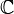The Burning Ship fractal process is similar to the Mandelbrot Set process, but now the real and imaginary components of z are replaced by their absolute values (i.e. replace – with +):

zn+1 = (|Re(zn)| + i * |Im(zn)|)2 + c

Wikiepdia: The Burning Ship fractal, first described and created by Michael Michelitsch and Otto E. Rössler in 1992, is generated by iterating the function:$z_{n+1} = (|\operatorname{Re} \left(z_n\right)|+i|\operatorname{Im} \left(z_n\right)|)^2 + c, \quad z_0=0$

in the complex plane$\mathbb{C}$ which will either escape or remain bounded. The difference between this calculation and that for theMandelbrot set is that the real and imaginary components are set to their respective absolute values before squaring at each iteration. The mapping is non-analytic because its real and imaginary parts do not obey the Cauchy–Riemann equations.

This site uses Akismet to reduce spam. Learn how your comment data is processed.